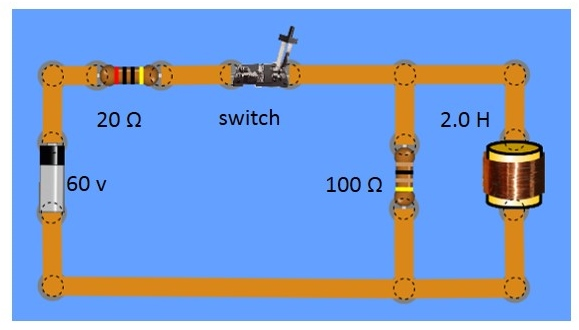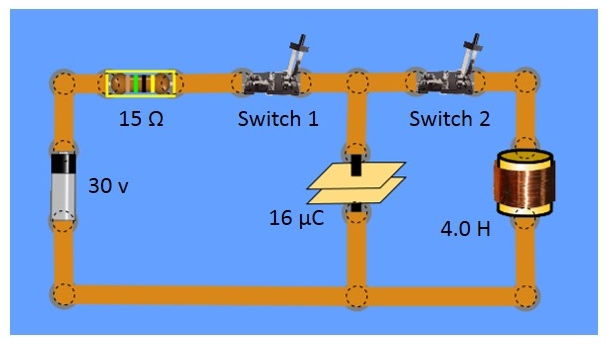Physics C Electricity and Magnetism
Click here to see the unit menu
Return to the home page to log out

Do you have questions? Click here to access the class discussion forum.

Quiz #12C E&M

Answer the questions below and then submit them in the form at the bottom of this page to see your score.

Before you attempt this quiz, carefully review your notes for the section of the unit that immediately precedes it. Take the quiz without using your notes. You can use the equations sheet produced by the College Board. Click here to download it or print it out.

1.

A resistor-inductor circuit with a time constant of 3.4 seconds has a total inductance of 450 H. What amount of resistance is in the circuit?

1. 1530 Ω
2. 153 Ω
3. 132 Ω
4. 74 Ω

2.

In the circuit described in question #1, a voltage source is used to power the circuit such that the rate of change in current with time is 0.034 A/s at a particular instant. What is the potential difference across the inductor at that moment?

1. 15.3 v
2. 13235 v
3. 4.5 v
4. 9.0 v

3.

Refer once again to the circuit from question #1. If the energy stored in the inductor is 5.2 J at steady state, what is the maximum current?

1. 0.15 A
2. 0.72 A
3. 0.31 A
4. 0.44 A

4.

Analyze the RL circuit below to determine the current in the inductor the moment the switch is closed.1. 0 A
2. 3.0 A
3. 0.5 A
4. 1.0 A

5.

Refer to the circuit in the previous question. What is the rate at which the current changes immediately after the switch is closed?

1. 10 A/s
2. 2 A/s
3. 25 A/s
4. 50 A/s

6.

Consider the circuit shown below. Switch 1 is closed until the capacitor reaches steady state. At that point, switch 1 opens. Subsequently, switch 2 closes. Which of the following is true?1. A maximum current will immediately occur
2. The energy stored in the capacitor will remain constant
3. The current through the inductor is initially zero
4. The capacitor will remain in steady state for a while

7.

Calculate the oscillation frequency for the circuit in question #6 after switch 2 closes.

1. 43.0 Hz
2. 81 Hz
3. 19.9 Hz
4. 5.8 Hz

8.

Calculate the maximum current for the circuit in question 6 after switch 2 is closed.

1. 0.03 A
2. 0.06 A
3. 0.09 A
4. 0.12 A

9.

An RL circuit with time constant τ effectively reaches steady state after 60 seconds. If the resistance and the inductance in the circuit both double, how will that affect the time to effectively reach steady state?

1. The time needed will effectively double to 120 seconds
2. The time needed will effectively half to 30 seconds
3. The time needed will not change
4. The time needed will effectively quadruple

10.

An LC circuit with L = 0.003 H and C = 0.001 F has a maximum charge on the capacitor at time t = 0 on a timer. What time is measured to have the voltage across the capacitor completely reverse for the first time?

1. 8.2 ms
2. 2.7 ms
3. 10.8 ms
4. 5.4 ms

Powered by Physics Prep LLC.  All rights reserved. ©2012-2020